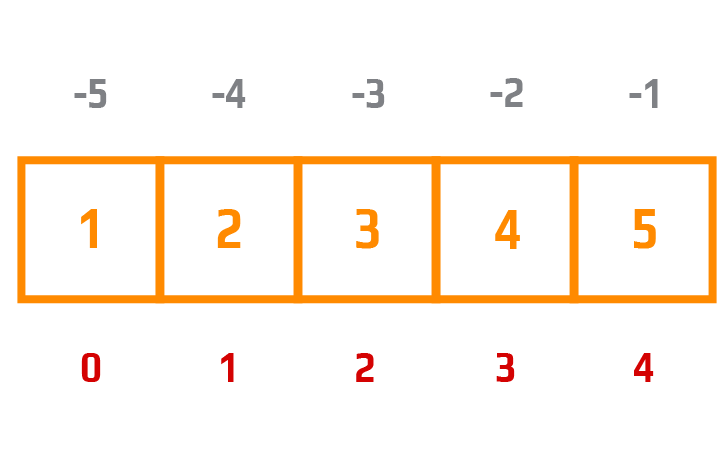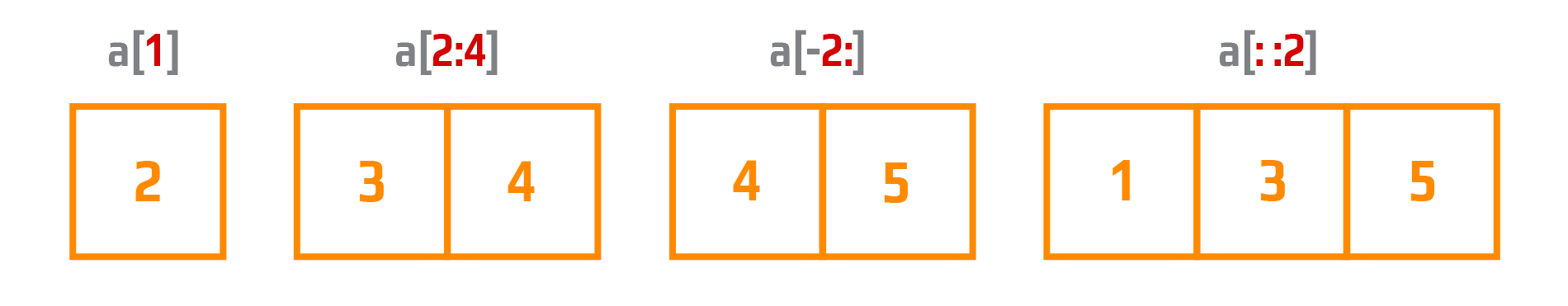Course Content

# NumPy in a Nutshell

NumPy in a Nutshell

##Access Array Elements

Both in lists and in arrays, elements are accessed using regular square brackets. Let's remember the difference between indexing and slicing.

• In order to get a single element, we just need to specify the index of this element in square brackets (start counting from 0).
• Therefore, in order to obtain a sequence from the original array, we should use slices.

We start with a simple indexing. Let's look at the next image.Let's see how it works with examples.

Get the first element from the following array:Get the second element from the following array:Get the third and the fourth elements from the following array and add them:Now it's time to look at using slicing. First, let's look at the syntax of slicing: `array[start:end:step]` respectively:

• start - the index from which to start slicing.
• end - the index till which the slicing ends (! this index is not included).
• step - this parameter determines the increments between the indices.

Let's look at the next image.It's time to practice.

Get the first and the last elements from the following array `[13, 99, 11, 23, 4, 41]` and multiply them. Please, use positive indexing.